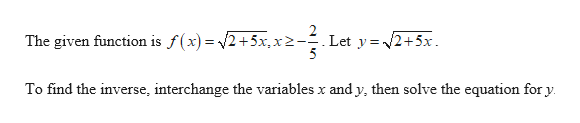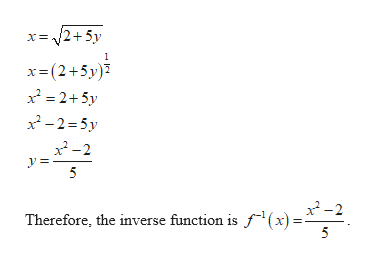# Find the inverse function f-1(x) of the function. Also, state the range of the inverse function in interval notation.f(x)= the square root of (2+5x) , x greater than or equal to -2/5

Question
2 views

Find the inverse function f-1(x) of the function. Also, state the range of the inverse function in interval notation.

f(x)= the square root of (2+5x) , x greater than or equal to -2/5

check_circle

Step 1help_outlineImage TranscriptioncloseThe given function is f(x) = 2+5x,x2- Let y = /2+5x. To find the inverse, interchange the variables x and y, then solve the equation for y. fullscreen
Step 2help_outlineImage Transcriptionclosex= /2+5y x=(2+5y)7 x = 2+5y x²-2=5y x -2 5 Therefore, the inverse function is f(x) = fullscreen

### Want to see the full answer?

See Solution

#### Want to see this answer and more?

Solutions are written by subject experts who are available 24/7. Questions are typically answered within 1 hour.*

See Solution
*Response times may vary by subject and question.
Tagged in

### Math